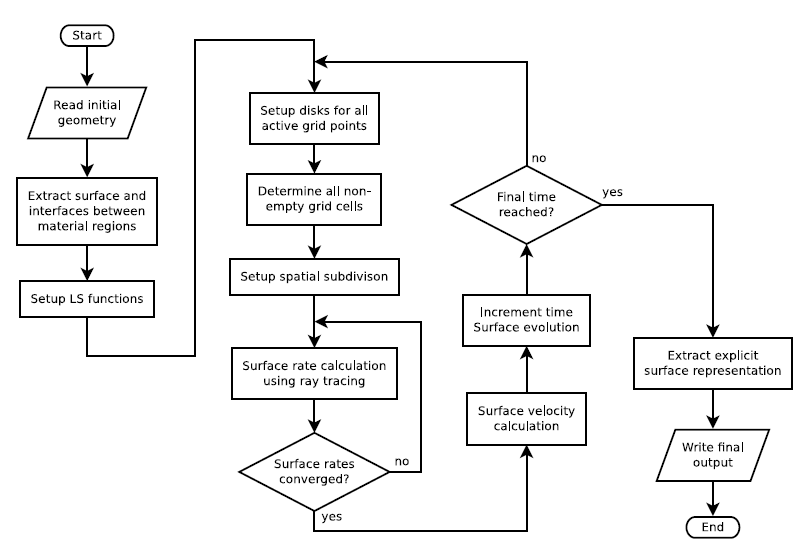Next: 5.4.2 Model Implementation Up: 5.4 Implementation Details Previous: 5.4 Implementation Details

## 5.4.1 Simulation Flow

The basic flow of the complete algorithm for topography simulation is depicted in Figure 5.7. First, the initial geometry is given as a volume mesh, where a material number is assigned to each volume element, which is either a triangle or a tetrahedron for two or three dimensions, respectively. The order of these numbers defines, in which way the structure is represented by LSs (see Section 4.6.1). The surface and the corresponding interfaces are then extracted, which results, depending on the number of dimensions, in triangle or line segmentations which are further transformed into LS representations.After initialization, the main cycle of the algorithm is started. For all active grid points the tangential disks are set up. For each active grid point the distance to the disk and the normal vector are calculated and stored. Afterwards, the set of all non-empty grid cells is determined, which is then used to set up an appropriate spatial subdivision. The subsequent ray tracing procedure calculates the surface rates which are needed to calculate the surface velocities.

As previously discussed in Section 2.2.3, the reemission probability of particles might depend on the arriving flux distribution, which is the case for the non-linear surface reactions presented in Section 2.3.2. This leads to a recursive problem which can be solved through iterations. Hence, the computation of the surface rates must be repeated several times, until convergence is achieved. In practice, this is usually performed only for the first time step. Later, the reemission probability is estimated from the surface rates, which have been obtained in the preceding time step. This is a reasonable approach, since subsequent geometric changes are very small due to the CFL condition.

After the calculation of the surface rates, the surface velocity can be computed for all active grid points. Then, the surface is advanced using the LS algorithm and the time is incremented. If the elapsed time is still smaller than the total processing time, the entire time evolution cycle is repeated. Otherwise, the marching squares or marching cubes algorithm, depending on the number of dimensions, is applied in order to obtain an explicit surface representation as final output.Next: 5.4.2 Model Implementation Up: 5.4 Implementation Details Previous: 5.4 Implementation Details

Otmar Ertl: Numerical Methods for Topography Simulation